# Selina Solutions Concise Mathematics Class 6 Chapter 2 Estimation Exercise 2(B)

Estimation of numbers with its illustrations is the main concept discussed under Exercise 2(B) of Selina Solutions Concise Mathematics Class 6 Chapter 2 Estimation. Our subject experts have solved these solutions with the intention to help students in solving the problems with ease. Students who fail to grasp during class hours are suggested to practice these solutions in-depth for better understanding. These solutions are the best study materials for students who aspire to score good marks in the examination. Students can make use of the PDF of Selina Solutions Concise Mathematics Class 6 Chapter 2 Estimation Exercise 2(B) and download free, from the links given below.

## Selina Solutions Concise Mathematics Class 6 Chapter 2 Estimation Exercise 2(B) Download PDF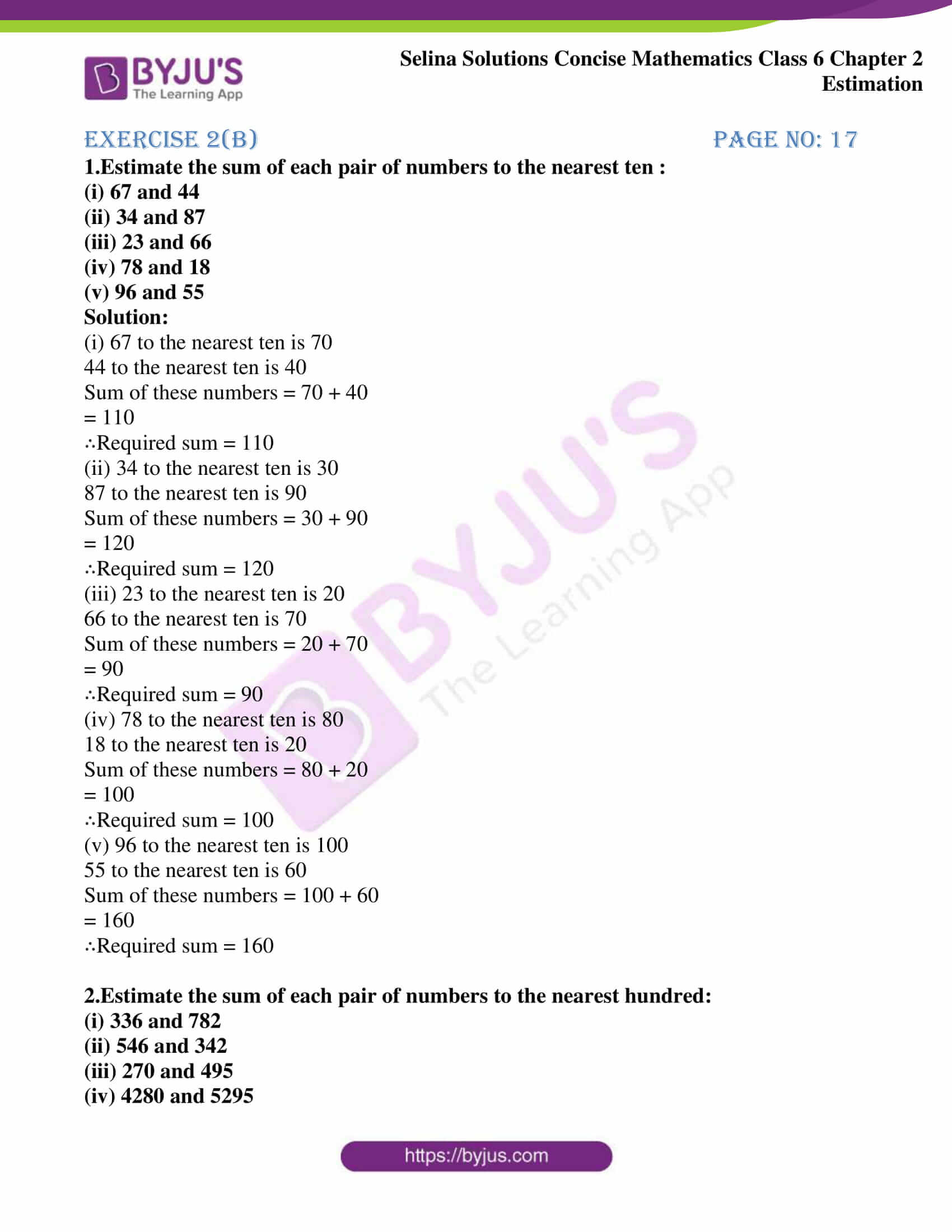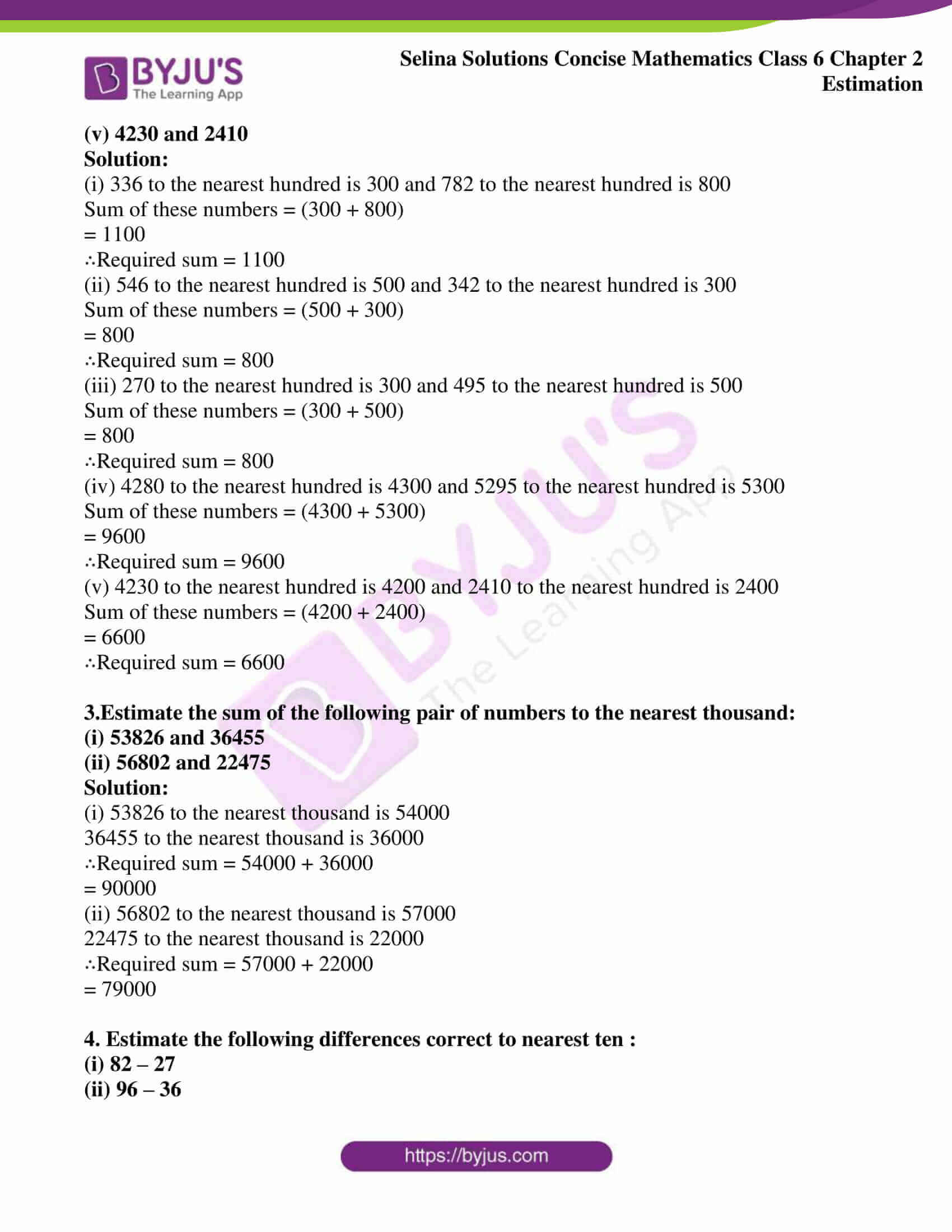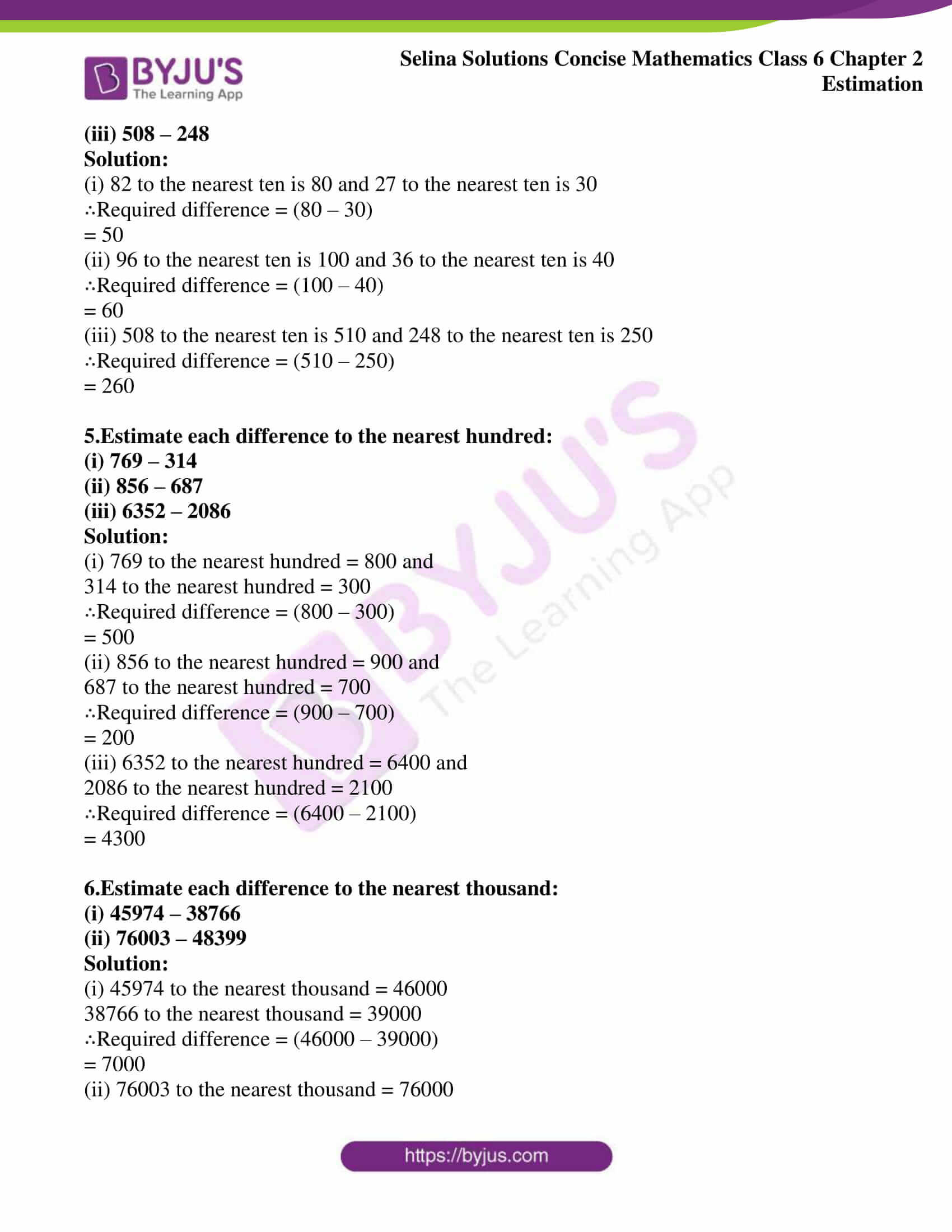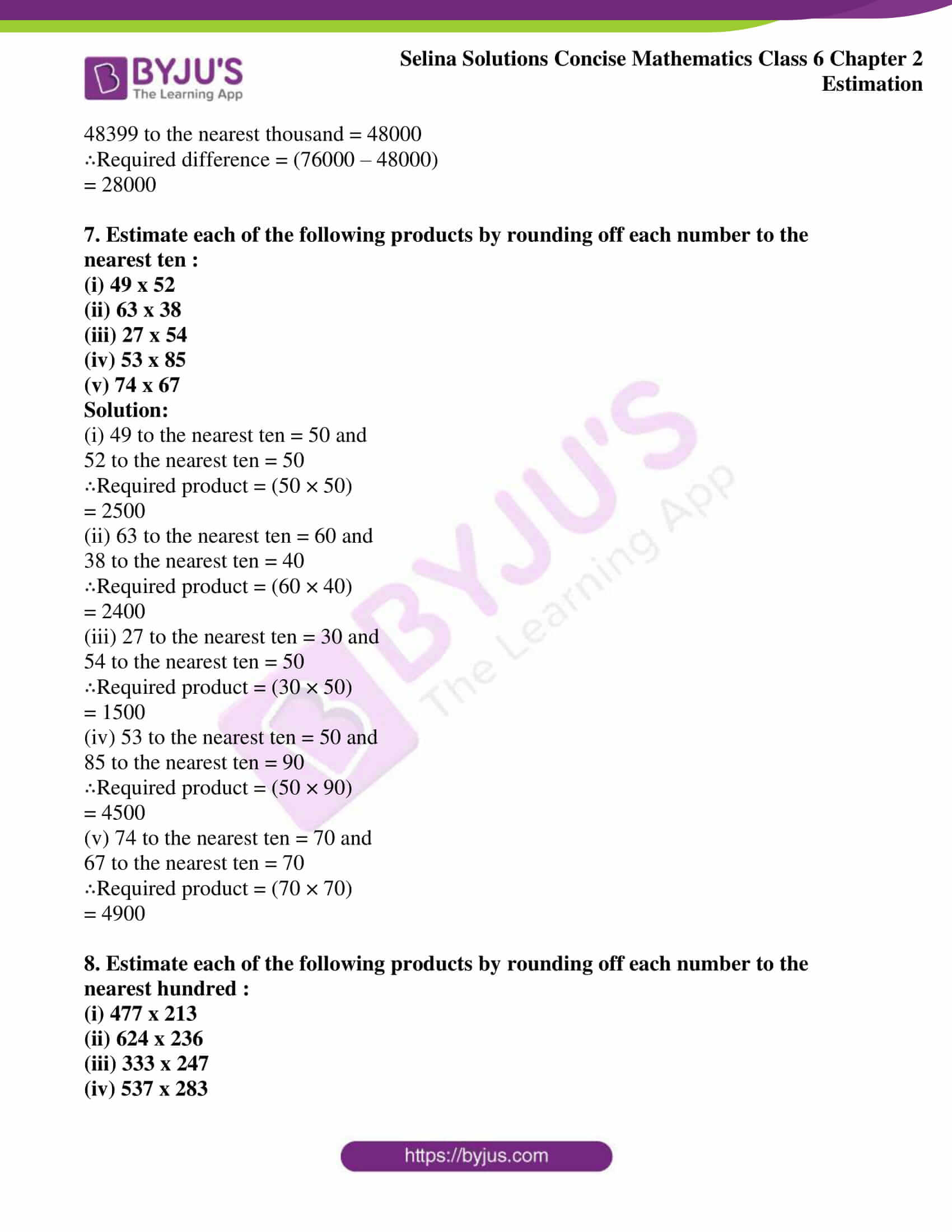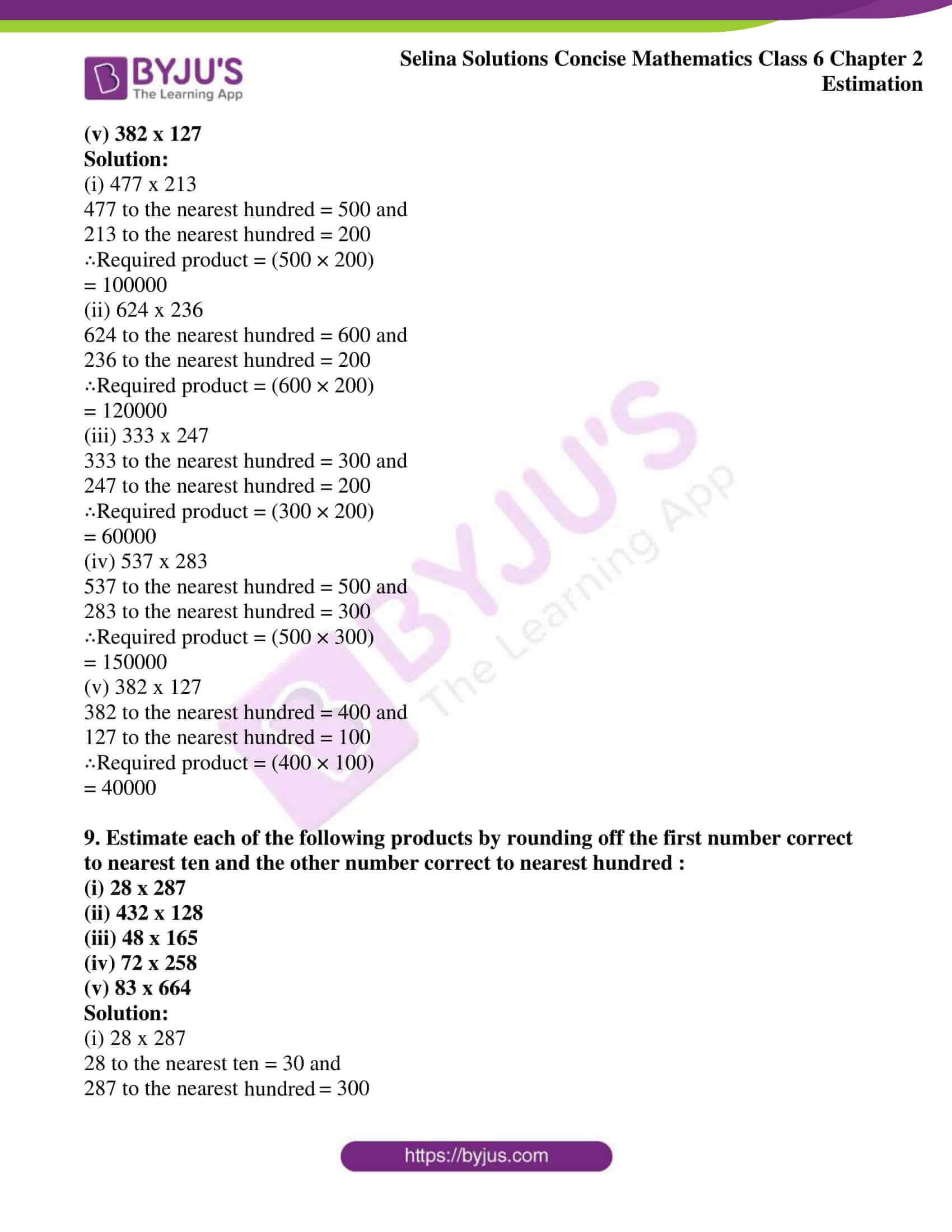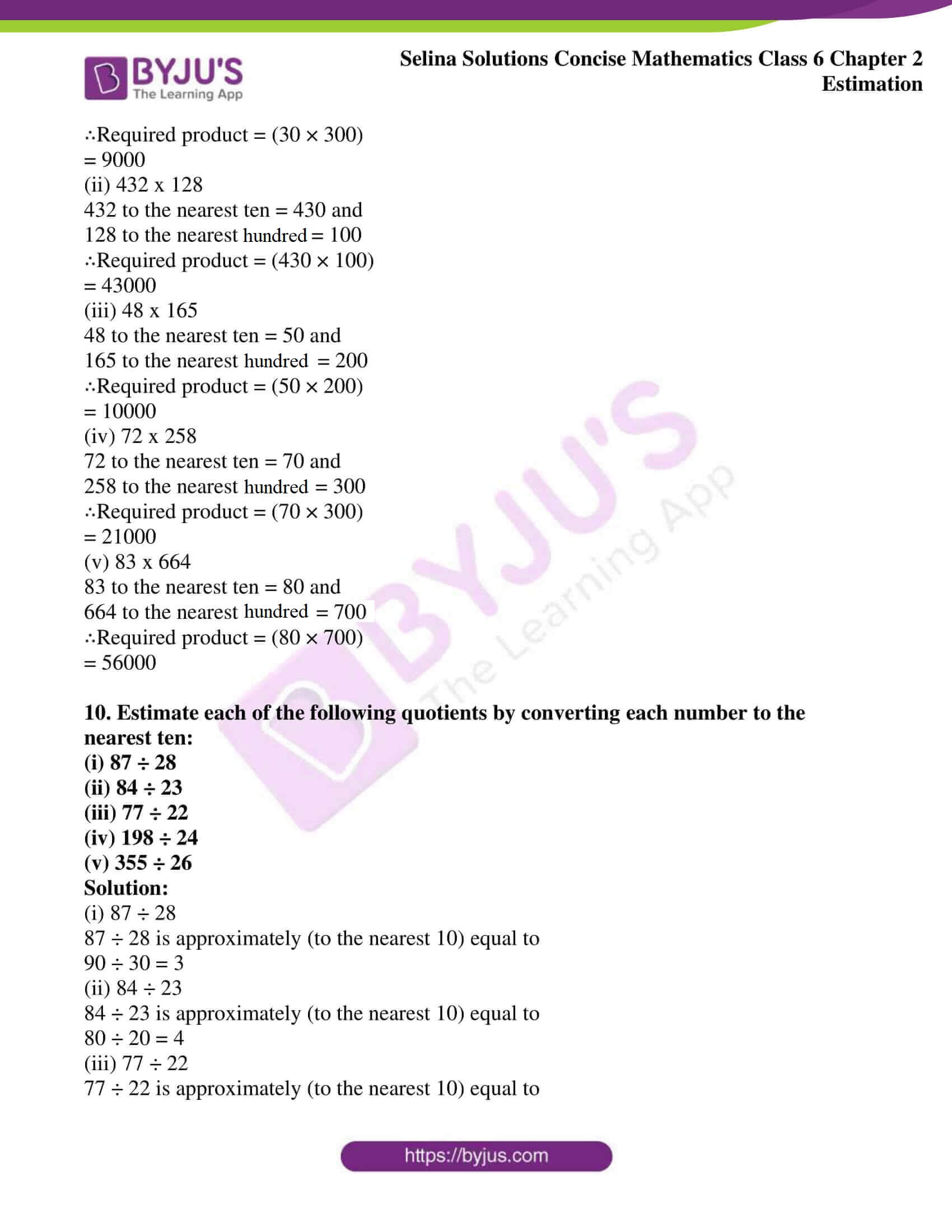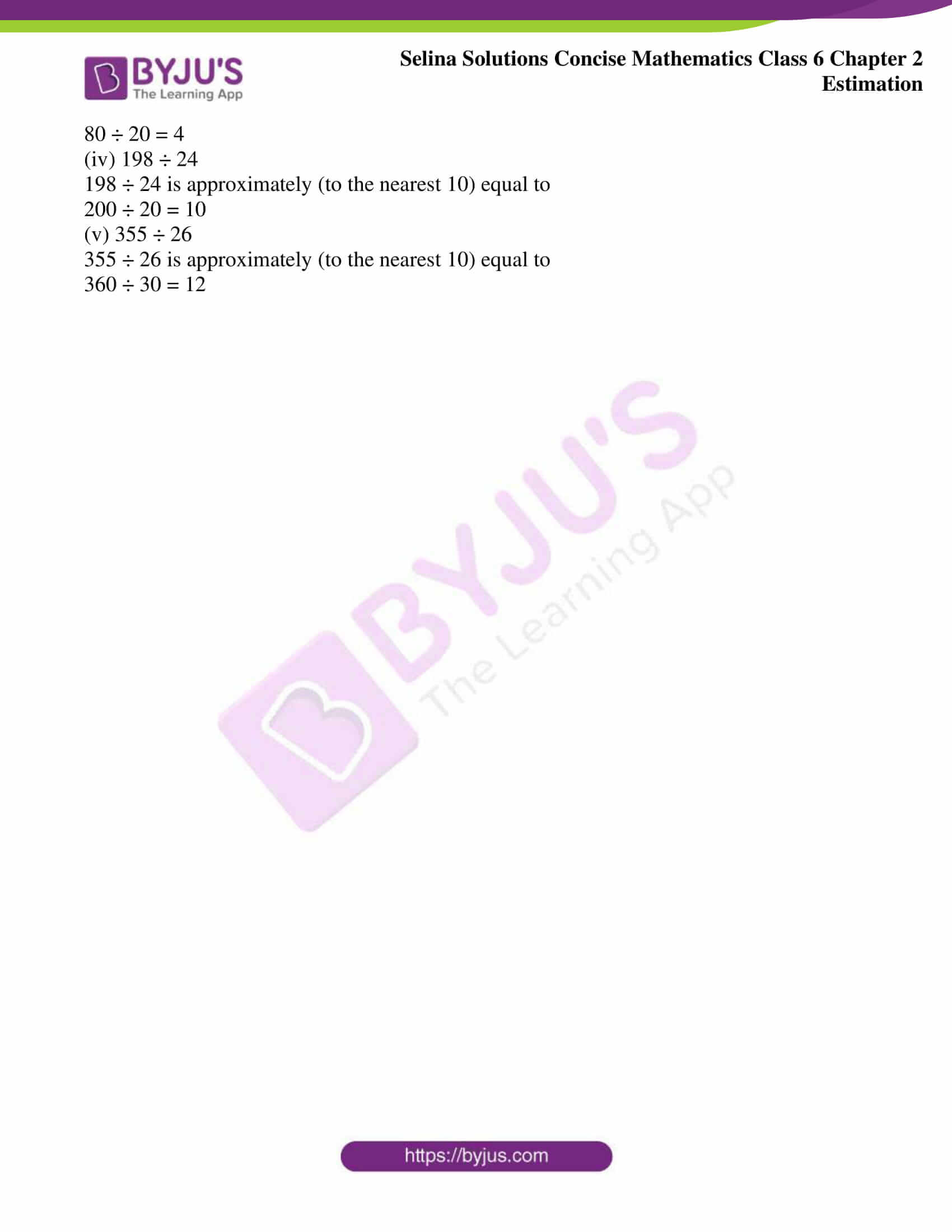### Access another exercise of Selina Solutions Concise Mathematics Class 6 Chapter 2 Estimation

Exercise 2(A) Solutions

### Access Selina Solutions Concise Mathematics Class 6 Chapter 2: Estimation Exercise 2(B)

#### Exercise 2(b) page no: 17

1.Estimate the sum of each pair of numbers to the nearest ten :
(i) 67 and 44
(ii) 34 and 87
(iii) 23 and 66
(iv) 78 and 18
(v) 96 and 55

Solution:

(i) 67 to the nearest ten is 70

44 to the nearest ten is 40

Sum of these numbers = 70 + 40

= 110

∴Required sum = 110

(ii) 34 to the nearest ten is 30

87 to the nearest ten is 90

Sum of these numbers = 30 + 90

= 120

∴Required sum = 120

(iii) 23 to the nearest ten is 20

66 to the nearest ten is 70

Sum of these numbers = 20 + 70

= 90

∴Required sum = 90

(iv) 78 to the nearest ten is 80

18 to the nearest ten is 20

Sum of these numbers = 80 + 20

= 100

∴Required sum = 100

(v) 96 to the nearest ten is 100

55 to the nearest ten is 60

Sum of these numbers = 100 + 60

= 160

∴Required sum = 160

2.Estimate the sum of each pair of numbers to the nearest hundred:
(i) 336 and 782
(ii) 546 and 342
(iii) 270 and 495
(iv) 4280 and 5295

(v) 4230 and 2410

Solution:

(i) 336 to the nearest hundred is 300 and 782 to the nearest hundred is 800

Sum of these numbers = (300 + 800)

= 1100

∴Required sum = 1100

(ii) 546 to the nearest hundred is 500 and 342 to the nearest hundred is 300

Sum of these numbers = (500 + 300)

= 800

∴Required sum = 800

(iii) 270 to the nearest hundred is 300 and 495 to the nearest hundred is 500

Sum of these numbers = (300 + 500)

= 800

∴Required sum = 800

(iv) 4280 to the nearest hundred is 4300 and 5295 to the nearest hundred is 5300

Sum of these numbers = (4300 + 5300)

= 9600

∴Required sum = 9600

(v) 4230 to the nearest hundred is 4200 and 2410 to the nearest hundred is 2400

Sum of these numbers = (4200 + 2400)

= 6600

∴Required sum = 6600

3.Estimate the sum of the following pair of numbers to the nearest thousand:
(i) 53826 and 36455
(ii) 56802 and 22475

Solution:

(i) 53826 to the nearest thousand is 54000

36455 to the nearest thousand is 36000

∴Required sum = 54000 + 36000

= 90000

(ii) 56802 to the nearest thousand is 57000

22475 to the nearest thousand is 22000

∴Required sum = 57000 + 22000

= 79000

4. Estimate the following differences correct to nearest ten :
(i) 82 – 27
(ii) 96 – 36
(iii) 508 – 248

Solution:

(i) 82 to the nearest ten is 80 and 27 to the nearest ten is 30

∴Required difference = (80 – 30)

= 50

(ii) 96 to the nearest ten is 100 and 36 to the nearest ten is 40

∴Required difference = (100 – 40)

= 60

(iii) 508 to the nearest ten is 510 and 248 to the nearest ten is 250

∴Required difference = (510 – 250)

= 260

5.Estimate each difference to the nearest hundred:
(i) 769 – 314
(ii) 856 – 687
(iii) 6352 – 2086

Solution:

(i) 769 to the nearest hundred = 800 and

314 to the nearest hundred = 300

∴Required difference = (800 – 300)

= 500

(ii) 856 to the nearest hundred = 900 and

687 to the nearest hundred = 700

∴Required difference = (900 – 700)

= 200

(iii) 6352 to the nearest hundred = 6400 and

2086 to the nearest hundred = 2100

∴Required difference = (6400 – 2100)

= 4300

6.Estimate each difference to the nearest thousand:
(i) 45974 – 38766
(ii) 76003 – 48399

Solution:

(i) 45974 to the nearest thousand = 46000

38766 to the nearest thousand = 39000

∴Required difference = (46000 – 39000)

= 7000

(ii) 76003 to the nearest thousand = 76000

48399 to the nearest thousand = 48000

∴Required difference = (76000 – 48000)

= 28000

7. Estimate each of the following products by rounding off each number to the nearest ten :
(i) 49 x 52
(ii) 63 x 38
(iii) 27 x 54
(iv) 53 x 85
(v) 74 x 67

Solution:

(i) 49 to the nearest ten = 50 and

52 to the nearest ten = 50

∴Required product = (50 × 50)

= 2500

(ii) 63 to the nearest ten = 60 and

38 to the nearest ten = 40

∴Required product = (60 × 40)

= 2400

(iii) 27 to the nearest ten = 30 and

54 to the nearest ten = 50

∴Required product = (30 × 50)

= 1500

(iv) 53 to the nearest ten = 50 and

85 to the nearest ten = 90

∴Required product = (50 × 90)

= 4500

(v) 74 to the nearest ten = 70 and

67 to the nearest ten = 70

∴Required product = (70 × 70)

= 4900

8. Estimate each of the following products by rounding off each number to the nearest hundred :
(i) 477 x 213
(ii) 624 x 236
(iii) 333 x 247
(iv) 537 x 283

(v) 382 x 127

Solution:

(i) 477 x 213

477 to the nearest hundred = 500 and

213 to the nearest hundred = 200

∴Required product = (500 × 200)

= 100000

(ii) 624 x 236

624 to the nearest hundred = 600 and

236 to the nearest hundred = 200

∴Required product = (600 × 200)

= 120000

(iii) 333 x 247

333 to the nearest hundred = 300 and

247 to the nearest hundred = 200

∴Required product = (300 × 200)

= 60000

(iv) 537 x 283

537 to the nearest hundred = 500 and

283 to the nearest hundred = 300

∴Required product = (500 × 300)

= 150000

(v) 382 x 127

382 to the nearest hundred = 400 and

127 to the nearest hundred = 100

∴Required product = (400 × 100)

= 40000

9. Estimate each of the following products by rounding off the first number correct to nearest ten and the other number correct to nearest hundred :
(i) 28 x 287
(ii) 432 x 128
(iii) 48 x 165
(iv) 72 x 258
(v) 83 x 664

Solution:

(i) 28 x 287

28 to the nearest ten = 30 and

287 to the nearest hundred = 300

∴Required product = (30 × 300)

= 9000

(ii) 432 x 128

432 to the nearest ten = 430 and

128 to the nearest hundred = 100

∴Required product = (430 × 100)

= 43000

(iii) 48 x 165

48 to the nearest ten = 50 and

165 to the nearest hundred = 200

∴Required product = (50 × 200)

= 10000

(iv) 72 x 258

72 to the nearest ten = 70 and

258 to the nearest hundred = 300

∴Required product = (70 × 300)

= 21000

(v) 83 x 664

83 to the nearest ten = 80 and

664 to the nearest hundred = 700

∴Required product = (80 × 700)

= 56000

10. Estimate each of the following quotients by converting each number to the nearest ten:
(i) 87 ÷ 28
(ii) 84 ÷ 23
(iii) 77 ÷ 22
(iv) 198 ÷ 24
(v) 355 ÷ 26

Solution:

(i) 87 ÷ 28

87 ÷ 28 is approximately (to the nearest 10) equal to

90 ÷ 30 = 3

(ii) 84 ÷ 23

84 ÷ 23 is approximately (to the nearest 10) equal to

80 ÷ 20 = 4

(iii) 77 ÷ 22

77 ÷ 22 is approximately (to the nearest 10) equal to

80 ÷ 20 = 4

(iv) 198 ÷ 24

198 ÷ 24 is approximately (to the nearest 10) equal to

200 ÷ 20 = 10

(v) 355 ÷ 26

355 ÷ 26 is approximately (to the nearest 10) equal to

360 ÷ 30 = 12